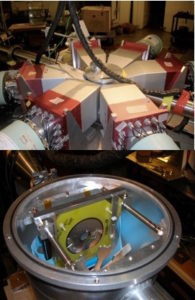Stellar nucleosynthesis processes involve thousands of reactions for which the cross sections need to be described using theoretical models. For a wide variety of processes, e.g. p-, s- and r- process the nucleosynthesis path runs through heavy nuclei, thus statistical Hauser-Feshbach (HF) models can be applied to describe the cross sections. HF models requires as input, among others, γ-strength (γSF) and level density (LD) functions, and optical model potential (OMP). A wide variety of models that describe these quantities (see e.g. TALYS code), results in cross sections predictions that can vary even by an order of magnitude, resulting in large uncertainties in network calculations of the nucleosynthesis processes.